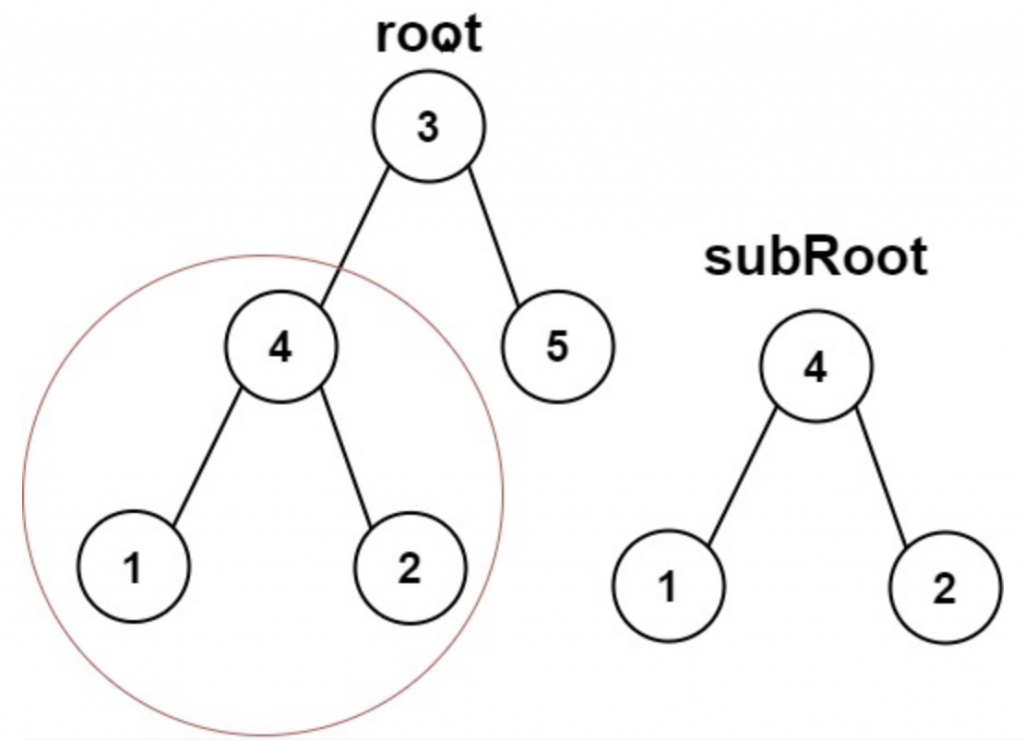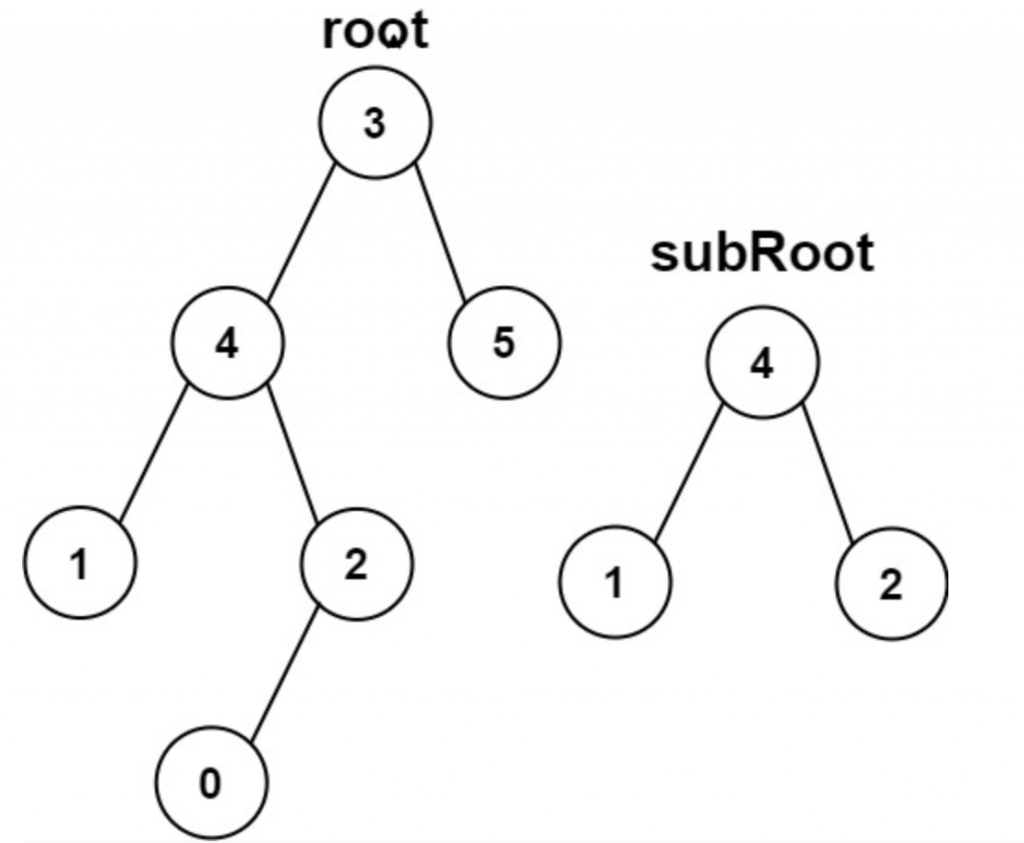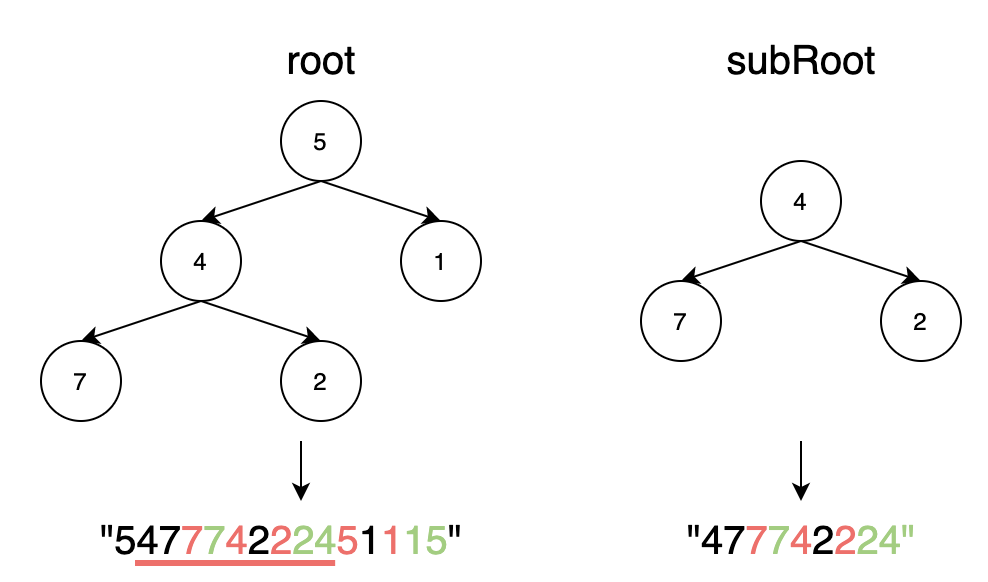DAY 27
0
Software Development

簡單說說 Hash Function

• Hash 過後得到的值是有可能遇到碰撞的問題，也就是說兩個不一樣的資料 Hash 過後卻得到相同的值，這樣就是所謂的碰撞，在設計 Hash Function 時需要特別小心。
• Hash 過後得到的值，在不知道 Hash Function 邏輯的狀況下，理論上是很難推回到原本資料的長相。

題目解說

• root 的節點總數範圍在[1, 2000].
• subRoot 的節點總數範圍在 [1, 1000].
• -10的4次方 <= root.val <= 10的4次方
• -10的4次方 <= subRoot.val <= 10的4次方解題的想像

文字描述

1. 寫一個方法將樹轉成字串。
2. 將題目給的 root 及 subRoot 轉成字串。
3. 若 subRoot 的字串有出現在 root 的字串中，代表這顆 subRoot 的樹有出現在 root 中，回True，相反的沒出現就回 False。

圖解過程

root = [5, 4, 1, 7, 2]
subRoot = [4, 7, 2]程式碼撰寫

class Solution:
def convertString(self, root, rootStr = ''):
if root == None: return rootStr

rootStr += str(root.val)
rootStr = self.convertString(root.left, rootStr)
rootStr += str(root.val)
rootStr = self.convertString(root.right, rootStr)
rootStr += str(root.val)

return rootStr

def isSubtree(self, root: Optional[TreeNode], subRoot: Optional[TreeNode]) -> bool:
subRootStr = self.convertString(subRoot)
rootStr = self.convertString(root)

return subRootStr in rootStr

希望您今天能瞭解到...

1. Hash Function 的基本概念
2. 572. Subtree of Another Tree 的解題方法

Next：1. Two Sum